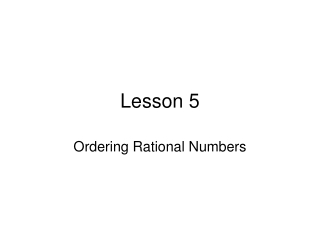DownloadDownload PresentationLesson 5

# Lesson 5

Download Presentation## Lesson 5

- - - - - - - - - - - - - - - - - - - - - - - - - - - E N D - - - - - - - - - - - - - - - - - - - - - - - - - - -
##### Presentation Transcript

1. Lesson 5 Ordering Rational Numbers

2. Putting numbers in order is easy! • Sometimes, you need to compare and order rational numbers. If the numbers are in different forms, you will need to put them into the same form before you can start to compare them. • Different forms? Well some numbers are written as fractions, some as decimals and some percents! What do you do?!

3. Ok, so what do I do?! • Let’s practice. Put these numbers in order from least to greatest: 0.2, -1/10, |-3/2|. 60%, √4/25 • We need to pick a form to change the numbers into. Let’s pick decimal. • 0.2 is already a decimal. • How do we change a fraction to a decimal?

4. Same problem • -1/10: Divide the top by the bottom: -1÷ 10= -0.1 • |-3/2|= 3/2, now we do the same as before: 3÷2= 1.5 • 60%: percents are a number out of 100, so 60 ÷100= .6 (it is the same as moving the decimal point 2 places to the left). • √4/25. √4=2 and √25=5. Now we have 2/5. 2 ÷5= .4

5. Almost done • So now we have: 0.2, -0.1, 1.5, 0.6 and 0.4. • Put them in order from least to greatest: -0.1, 0.2, 0.4, 0.6, 1.5. • The only thing we need to do is change them back to what they were before. • Final answer: -1/10, 0.2,√4/25, 60%, |-3/2|.

6. Let’s try some more • Which number has the least value? ¾, √16/81, 0.74, 80% • Which form do you change them to? Why? • If these were graphed on a number line, which would be closet to zero? 0.25, 1/20, 0.1, 3/10 • What is the strategy?

7. On your own • Order the numbers from least to greatest: 0.6, 4/5, 70% • Which is greater, ½ or √9/36? • Order the numbers from greatest to least: 5, -6, 0, |-1| • Which number would be third on a list if they were ordered from least to greatest: 100%, 3/8, 1/3, |-0.55|

8. The open ended • On a number line, plot points for the following numbers: 0.3, 14/20 • Label the points • Name two different rational numbers that are greater than 0.3 and less than 14/20. (Write one number as a fraction and the other as a decimal) • Explain how you know that each of the numbers you chose is greater than 0.3 and less than 14/20.